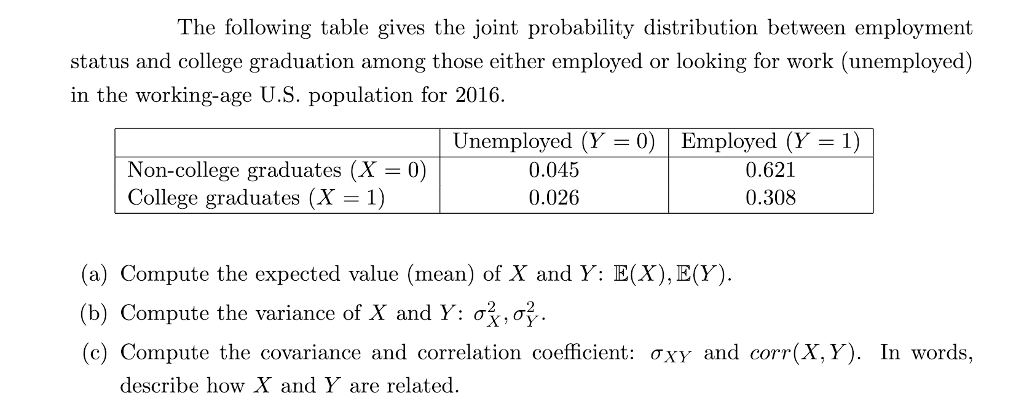# The following table gives the joint probability distribution between employment status and college graduation among those...

###### Question:The following table gives the joint probability distribution between employment status and college graduation among those either employed or looking for work (unemployed) in the working-age U.S. population for 2016. | Unemployed (Y = 0) . Employed (Y = 1) Non-college graduates (X- 0 College graduates (X 1) 0.045 0.026 0.621 0.308 (a) Compute the expected value (mean) of X and Y: E(X), E(Y). (b) Compute the variance of X and Y: ơ (c) Compute the covariance and correlation coefficient: ơXY and corr(X,Y). In words, describe how X and Y are related

#### Similar Solved Questions

##### Problem I A series of two-point crosses were carried out among seven loci (a, b, c,...
Problem I A series of two-point crosses were carried out among seven loci (a, b, c, d, e, f, and g), producing the following recombination frequencies. Map the seven loci, showing their linkage groups, the order of the loci in each linkage group, and distances between the loci of each linkage group....